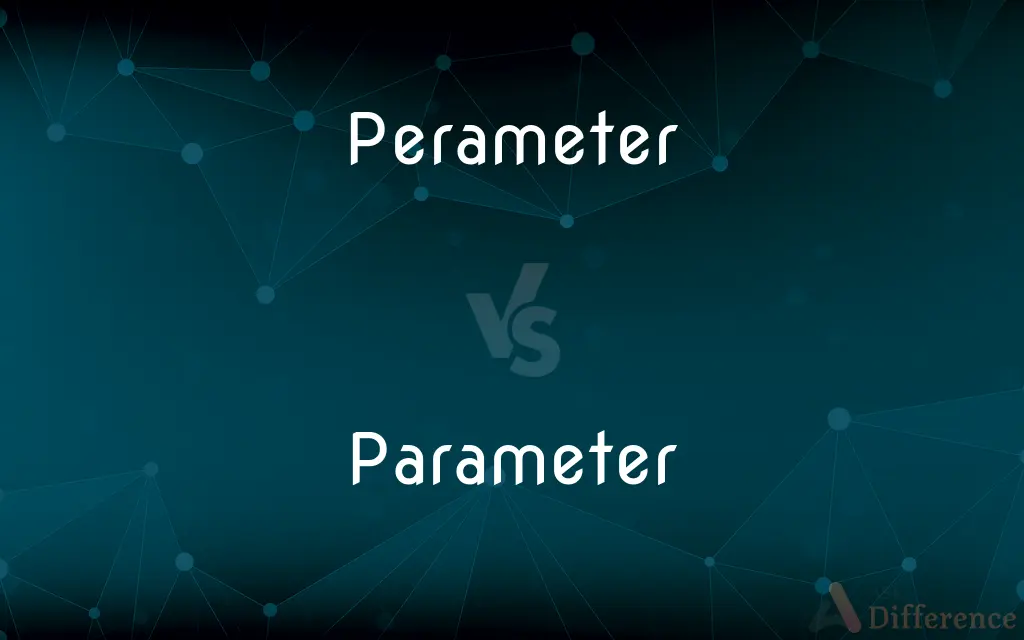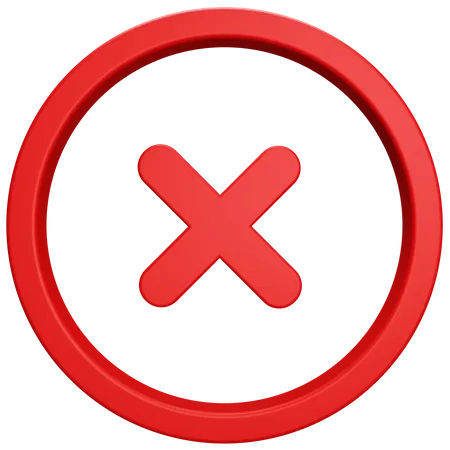# Perameter vs. Parameter — What's the Difference?

"Perameter" is an incorrect spelling. The correct spelling is "Parameter," which refers to a defining or limiting factor or element.## Which is correct: Perameter or Parameter

How to spell Parameter?### Perameter

Incorrect SpellingCorrect Spelling

## Key Differences

Recall "meter" in "parameter," as in measuring something.
Think of "para-" prefix meaning alongside or subsidiary.
Associate "parameter" with "perimeter" as they sound similar but have different meanings.
Visualize "para" as a pair, indicating two or alongside.
Remember it as "para" + "meter" (beside measure).

## Compare with Definitions

#### Perameter

Perameter is an incorrect spelling of Parameter.

#### Parameter

A distinguishing characteristic or feature.
One of the parameters of this classification system is size.

#### Parameter

A piece of data provided to a method or function in computer programming.
The function accepts two parameters.

#### Parameter

An element influencing behavior or results.
Social parameters affect consumer choices.

#### Parameter

A constant in an equation that varies in other equations of the same general form, especially such a constant in the equation of a curve or surface that can be varied to represent a family of curves or surfaces.

#### Parameter

One of a set of independent variables that express the coordinates of a point.

#### Parameter

One of a set of measurable factors, such as temperature and pressure, that define a system and determine its behavior and are varied in an experiment.

#### Parameter

Usage Problem A factor that restricts what is possible or what results
"all the parameters of shelter—where people will live, what mode of housing they will choose, and how they will pay for it" (New York).

#### Parameter

A factor that determines a range of variations; a boundary
An experimental school that keeps expanding the parameters of its curriculum.

#### Parameter

(Statistics) A quantity, such as a mean, that is calculated from data and describes a population.

#### Parameter

Usage Problem A distinguishing characteristic or feature.

#### Parameter

A value kept constant during an experiment, equation, calculation, or similar, but varied over other versions of the experiment, equation, calculation, etc.

#### Parameter

(sciences) A variable that describes some system (material, object, event, etc.) or some aspect thereof.

#### Parameter

(statistics) Any measured quantity of a statistical population that summarises or describes an aspect of the population.

#### Parameter

(machine learning) A variable of a model that is trained by a machine learning algorithm.

#### Parameter

(programming) An input variable of a function definition, that gets an actual value (argument) at execution time.

#### Parameter

An actual value given to such a formal parameter.

#### Parameter

A characteristic or feature that distinguishes something from others.

#### Parameter

(geometry) In the ellipse and hyperbola, a third proportional to any diameter and its conjugate, or in the parabola, to any abscissa and the corresponding ordinate.
The parameter of the principal axis of a conic section is called the latus rectum.

#### Parameter

(crystallography) The ratio of the three crystallographic axes which determines the position of any plane.

#### Parameter

(crystallography) The fundamental axial ratio for a given species.

#### Parameter

A constant number which is part of a theory, function, or calculation, whose value is not determined by the form of the theory or equation itself, and may in some cases be arbitrary assigned.

#### Parameter

A term applied to some characteristic magnitude whose value, invariable as long as one and the same function, curve, surface, etc., is considered, serves to distinguish that function, curve, surface, etc., from others of the same kind or family.

#### Parameter

Any constant number which is required to calculate values of observed phenomena according to a theory, but the value of which must be determined by experiment, and cannot be calculated from the fundamental assumptions of the theory. In general, a theory which has a large number of parameters, though it may accurately predict experimental results, is considered as having less explanatory power and as being less esthetically pleasing than a theory with fewer parameters.

#### Parameter

The ratio of the three crystallographic axes which determines the position of any plane; also, the fundamental axial ratio for a given species.

#### Parameter

The limits, guidelines, or assumptions from within which an activity is carried out; as, new arrivals need to learn the parameters of the research in our department.

#### Parameter

A variable used in a calculation within a computer program which must be assigned a value before the calculation can be performed; as, let's plug in the parameters and see what the result is.

#### Parameter

A characteristic or element, especially one used as a criterion for evaluation or judgment; as, a useful parameter for determining efficiency.

#### Parameter

A constant in the equation of a curve that can be varied to yield a family of similar curves

#### Parameter

Any factor that defines a system and determines (or limits) its performance

#### Parameter

A quantity (such as the mean or variance) that characterizes a statistical population and that can be estimated by calculations from sample data

#### Parameter

A numerical or other measurable factor forming one of a set defining a system or set of conditions.
The machine operates within specific parameters.

#### Parameter

A boundary or limit.
This problem is outside the parameters of our study.

## Common Curiosities

#### Why is it called Parameter?

It's derived from the Greek "para-" (beside or subsidiary) and "metron" (measure), indicating a measure alongside something else.

#### What is the root word of Parameter?

The Greek word "metron" (meaning measure) is a root, with the prefix "para-" meaning alongside.

#### Which vowel is used before Parameter?

"A" can be used before "parameter" (e.g., "a parameter").

#### What is the singular form of Parameter?

The singular form is "parameter."

No, "parameter" is not an adverb.

#### What is the verb form of Parameter?

"Parameter" is a noun. There isn't a direct verb form.

#### What is the pronunciation of Parameter?

"Parameter" is pronounced as /pəˈræm.ɪ.tər/.

#### Which preposition is used with Parameter?

"Within" or "of" can be used with "parameter" depending on the context (e.g., "within the parameters," "parameter of").

#### Which article is used with Parameter?

Either "a" or "the" can be used, depending on context.

#### What is the plural form of Parameter?

The plural form is "parameters."

#### Which conjunction is used with Parameter?

No specific conjunction is tied to the word. It depends on the sentence structure and meaning.

#### Is Parameter a noun or adjective?

"Parameter" is a noun.

#### Is Parameter a vowel or consonant?

"Parameter" is a word, consisting of both vowels and consonants.

#### Is the Parameter term a metaphor?

No, but it can be used metaphorically in certain contexts.

#### Is Parameter a negative or positive word?

"Parameter" is neutral; it doesn't inherently have a positive or negative connotation.

#### Is Parameter a countable noun?

Yes, it can be counted (e.g., three parameters).

#### What is the second form of Parameter?

"Parameter" doesn't have verb forms, so there isn't a second form.

#### What is the third form of Parameter?

"Parameter" doesn't have verb forms, so there isn't a third form.

#### Is Parameter an abstract noun?

Yes, it can be considered an abstract noun as it represents a concept or idea rather than a tangible object.

#### Is the word Parameter imperative?

No, "parameter" is not imperative.

#### How do we divide Parameter into syllables?

It can be divided as Pa-ra-me-ter.

#### What is a stressed syllable in Parameter?

The second syllable "ra" is stressed.

#### What is the first form of Parameter?

"Parameter" doesn't have verb forms, so there isn't a first form.

#### How is Parameter used in a sentence?

"The study was conducted within the parameters of the scientific guidelines."

#### What is another term for Parameter?

Another term could be "criterion" or "factor."

#### Is Parameter a collective noun?

No, "parameter" is not a collective noun.

#### How many syllables are in Parameter?

"Parameter" has four syllables.

#### What part of speech is Parameter?

"Parameter" is a noun.

#### What is the opposite of Parameter?

There isn't a direct antonym, but "unlimited" or "unrestricted" might be used in contrast in certain contexts.

#### Which determiner is used with Parameter?

Determiners like "this," "that," "these," "those," "my," "our," etc. can be used with "parameter" based on context.